Midterm 2 Preparation

# Midterm 2: Version C

Find the solution set of the system graphically.

1.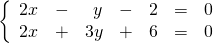For problems 2–4, find the solution set of each system by any convenient method.

1.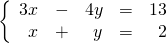2.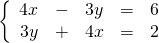3.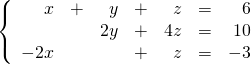Reduce the following expressions in questions 5–7.

1.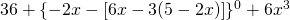2.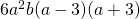3.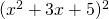Divide using long division.

1.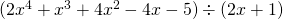For problems 9–12, factor each expression completely.

1.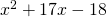2.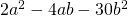3.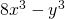4.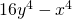Solve the following word problems.

1. The sum of a brother’s and sister’s ages is 30. Ten years ago, the brother was four times his sister’s age. How old are they now?
2. Kyra gave her brother Mark a logic question to solve: If she has 18 coins in her pocket worth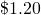, and if the coins are only dimes and nickels, how many of each type of coin does she have?
3. Tanya needs to make 10 litres of a 25% alcohol solution for the University Green College Founders Social by mixing a 30% alcohol solution with a 5% alcohol solution. How many litres each of the 30% and the 5% alcohol solutions should be used?